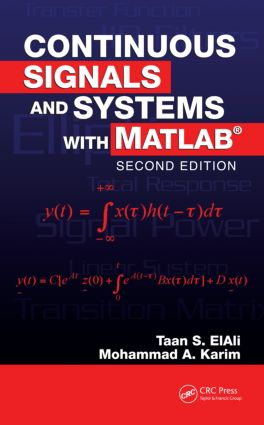# Continuous Signals and Systems with MATLAB

## 2nd Edition

CRC Press

520 pages | 152 B/W Illus.

##### Purchasing Options:\$ = USD
Hardback: 9781420054743
pub: 2008-01-17
SAVE ~\$28.00
Currently out of stock
\$140.00
\$112.00
x
eBook (VitalSource) : 9781315219356
pub: 2018-10-03
from \$70.00

FREE Standard Shipping!

### Description

Designed for a one-semester undergraduate course in continuous linear systems, Continuous Signals and Systems with MATLAB®, Second Edition presents the tools required to design, analyze, and simulate dynamic systems. It thoroughly describes the process of the linearization of nonlinear systems, using MATLAB® to solve most examples and problems. With updates and revisions throughout, this edition focuses more on state-space methods, block diagrams, and complete analog filter design.

New to the Second Edition

• A chapter on block diagrams that covers various classical and state-space configurations

• A completely revised chapter that uses MATLAB to illustrate how to design, simulate, and implement analog filters

• Numerous new examples from a variety of engineering disciplines, with an emphasis on electrical and electromechanical engineering problems

Explaining the subject matter through easy-to-follow mathematical development as well as abundant examples and problems, the text covers signals, types of systems, convolution, differential equations,Fourier series and transform, the Laplace transform, state-space representations, block diagrams, system linearization, and analog filter design. Requiring no prior fluency with MATLAB, it enables students to master both the concepts of continuous linear systems and the use of MATLAB to solve problems.

Preface

Signal Representation

Examples of Continuous Signals

The Continuous Signal

Periodic and Nonperiodic Signals

General Form of Sinusoidal Signals

Energy and Power Signals

The Shifting Operation

The Reflection Operation

Even and Odd Functions

Time Scaling

The Unit Step Signal

The Signum Signal

The Ramp Signal

The Sampling Signal

The Impulse Signal

Some Insights: Signals in the Real World

Continuous Systems

Definition of a System

Input and Output

Linear Continuous System

Time-Invariant System

Systems without Memory

Causal Systems

The Inverse of a System

Stable Systems

Convolution

Simple Block Diagrams

Graphical Convolution

Differential Equations and Physical Systems

Homogeneous Differential Equations and Their Solutions

Nonhomogeneous Differential Equations and Their Solutions

The Stability of Linear Continuous Systems:

The Characteristic Equation

Block Diagram Representation of Linear Systems

From Block Diagrams to Differential Equations

From Differential Equations to Block Diagrams

The Impulse Response

Some Insights: Calculating y(t)

Fourier Series

Review of Complex Numbers

Orthogonal Functions

Periodic Signals

Conditions for Writing a Signal as a Fourier Series Sum

Basis Functions

The Magnitude and the Phase Spectra

Fourier Series and the Sin-Cos Notation

Fourier Series Approximation and the Resulting Error

The Theorem of Parseval

Systems with Periodic Inputs

A Formula for Finding y(t) When x(t) Is Periodic: The Steady-State Response

Some Insight: Why the Fourier Series?

The Fourier Transform and Linear Systems

Definition

Introduction

The Fourier Transform Pairs

Energy of Nonperiodic Signals

The Energy Spectral Density of a Linear System

Some Insights: Notes and a Useful Formula

The Laplace Transform and Linear Systems

Definition

The Bilateral Laplace Transform

The Unilateral Laplace Transform

The Inverse Laplace Transform

Block Diagrams Using the Laplace Transform

Representation of Transfer Functions as Block Diagrams

Procedure for Drawing the Block Diagram from the Transfer Function

Solving LTI Systems Using the Laplace Transform

Solving Differential Equations Using the Laplace Transform

The Final Value Theorem

The Initial Value Theorem

Some Insights: Poles and Zeros

State-Space and Linear Systems

Introduction

A Review of Matrix Algebra

General Representation of Systems in State Space

General Solution of State-Space Equations Using the Laplace Transform

General Solution of the State-Space Equations in Real Time

Ways of Evaluating eAt

Some Insights: Poles and Stability

Block Diagrams

Introduction

Basic Block Diagram Components

Block Diagrams as Interconnected Subsystems

The Controllable Canonical Form Block Diagrams with Basic Blocks

The Observable Canonical Form Block Diagrams with Basic Blocks

The Diagonal Form Block Diagrams with Basic Blocks

The Parallel Block Diagrams with Subsystems

The Series Block Diagrams with Subsystems

Block Diagram Reduction Rules

Analog Filter Design

Introduction

Analogue Filter Specifications

Butterworth Filter Approximation

Chebyshev Filters

Elliptic Filter Approximation

Bessel Filters

Analog Frequency Transformation

Analog Filter Design Using MATLAB

How Do We Find the Cutoff Frequency Analytically?

Limitations

Comparison between Analog Filter Types

Implementation of Analog Filters

Some Insights: Filters with High Gain versus Filters with Low Gain and the Relation between the Time Constant and the Cutoff Frequency for First-Order Circuits and the Series RLC Circuit

Introduction to Nonlinear Systems

Introduction

Linear and Nonlinear Differential Equations

The Process of Linearization

Some Insights: The Meaning of Linear and Nonlinear

Index

Each chapter contains Examples, Problems, and References at the end.

### About the Originator

#### Dr. Taan ElAli

Columbia,, South Carolina,, USA